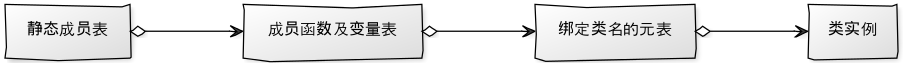# 我们的Lua类绑定机制

## 函数绑定的接口形式

// 这个FightBullet名字可以随意，只要保证全局唯一并且符合c++标识符规则即可，类似gtest
LUA_BIND_OBJECT(FightBullet) {
// 这里面的代码会在Lua绑定管理器初始化时自动执行
lua_State* L = script::lua::LuaEngine::Instance()->getLuaState();

// 声明一个类，命名空间是game.logic，类名是FightBullet
script::lua::LuaBindingClass<FightBullet> clazz("FightBullet", "game.logic", L);

// 绑定类成员函数或static函数，将会自动推断函数、返回值和参数类型
{
}
}


## 面向对象的实现原理

### Lua层面向对象(模拟继承和覆盖)

Lua原生并不支持面向对象设计,所以我们这里使用了一个简单的方法对面向对象的特性做一些模拟。

function gettable_event (table, key)
local h
if type(table) == "table" then
local v = rawget(table, key)
if v ~= nil then return v end
h = metatable(table).__index
if h == nil then return nil end
else
h = metatable(table).__index
if h == nil then
error(···)
end
end
if type(h) == "function" then
return (h(table, key))     -- call the handler
else return h[key]           -- or repeat operation on it
end
end


local father = {}
local child = {}
setmetatable(child, child)
child.__index = father


local father = {}
local child = {}
setmetatable(child, {__index = father})


-- tostring方法
function class.object:__tostring()
if self then
local ret = '[' .. self.__type .. ' : ' .. self.__classname .. ']'
if self.__instance_id then
ret = ret .. ' @' .. tostring(self.__instance_id)
end
return ret
end
return '[class : object] WARNING: you should use obj:__tostring but obj.__tostring'
end


### C++ binding层面向对象

C++类型绑定以后，也是走得上面的机制，所不一样的地方是，像诸如__tostring方法我们在本地（也就是C++）实现，并且把类型设为native code### 单例及命名空间

-- 单例基类
do
class.singleton = class.register('utils.class.singleton')
rawset(class.singleton, 'new', function(self, inst)
if nil == self then
log_error('singleton class must using class:new or class:instance(class.new and class.instance is unavailable)')
return nil
end
return self
end)

rawset(class.singleton, 'instance', class.singleton.new)
end


-- 命名空间
class.namespace = class.register('utils.class.namespace', class.singleton)


### 保护父类数据和const模拟

-- 保护table数据
function class.protect(obj, r)
local tmb = getmetatable(obj)
if tmb and class.readonly__newindex == tmb.__newindex then
obj = tmb.__index
end

local wrapper = {}
local wrapper_metatable = {}
setmetatable(wrapper, wrapper_metatable)

for k, v in pairs(obj) do
if r and 'table' == type(v) or 'userdata' == type(v) then
rawset(wrapper, k, class.protect(v, r))
else
rawset(wrapper, k, v)
end
end

rawset(wrapper_metatable, '__index', obj)
rawset(wrapper_metatable, '__newindex', function(tb, k, v)
rawset(tb, k, v)
end)
return wrapper
end


-- readonly 重载__newindex函数
log_error('table %s is readonly, set key %s is invalid', tostring(tb), tostring(key))
end

local tmb = getmetatable(obj)
if not tmb or class.readonly__newindex ~= tmb.__newindex then
local wrapper = {
__index = obj,
}

function wrapper:table_len()
return #obj
end

function wrapper:table_pairs()
return pairs(obj)
end

function wrapper:table_ipairs()
return ipairs(obj)
end

function wrapper:table_next(index)
return next(obj, index)
end

function wrapper:table_unpack(index)
return table.unpack(obj, index)
end

-- 原始table
function wrapper:table_raw()
return obj
end

-- 复制可写表
function wrapper:table_make_writable()
local ret = table.extend(obj)
for k, v in pairs(ret) do
if 'table' == type(v) then
rawset(ret, k, v:table_make_writable())
else
rawset(ret, k, v)
end
end

return ret
end

setmetatable(wrapper, wrapper)

for k, v in pairs(obj) do
if 'table' == type(v) or 'userdata' == type(v) then
end
end
return wrapper
end
return obj
end


## 对象的生命周期管理

local ut = unit.new(unit_id) -- 这里这个对象引用计数为1，在缓存池里。如果没有缓存池，引用计数为0，就会被销毁
-- ut 只有一次弱引用，不会影响实际的对象回收


## 函数类型和函数参数的自动判定

Lua绑定C++函数的时候，有可能出现各种函数类型。为了减少代码，我们大量使用了C++11的特性（主要是function、lambda表达式、type_traits和动态模板参数）。利用C++的模板参数推导规则来自动分析参数。比如成员函数：

/**
* 给类添加方法，自动推断类型
*
* @tparam  TF  Type of the tf.
* @param   func_name   Name of the function.
* @param   fn          The function.
*/
template<typename R, typename... TParam>
self_type& addMethod(const char* func_name, R(*fn)(TParam... param)) {
lua_State* state = getLuaState();
lua_pushstring(state, func_name);
lua_pushlightuserdata(state, reinterpret_cast<void*>(fn));
lua_pushcclosure(state, detail::unwraper_static_fn<R, TParam...>::LuaCFunction, 1);
lua_settable(state, getStaticClassTable());

return (*this);
}


/*************************************\
|* 静态函数Lua绑定，动态参数个数          *|
\*************************************/
template<typename Tr, typename... TParam>
struct unwraper_static_fn : public unwraper_static_fn_base<Tr> {
typedef unwraper_static_fn_base<Tr> base_type;
typedef Tr (*value_type)(TParam...);

// 动态参数个数
static int LuaCFunction(lua_State* L) {
value_type fn = reinterpret_cast<value_type>(lua_touserdata(L, lua_upvalueindex(1)));
if (nullptr == fn) {
// 找不到函数
return 0;
}

return base_type::template run_fn<value_type, std::tuple<
typename std::remove_cv<typename std::remove_reference<TParam>::type>::type...
> >(L,
fn,
typename build_args_index<TParam...>::index_seq_type()
);
}
};


template<int... _index>
struct index_seq_list{ typedef index_seq_list<_index..., sizeof...(_index)> next_type; };

template <typename... TP>
struct build_args_index;

template <>
struct build_args_index<>
{
typedef index_seq_list<> index_seq_type;
};

template <typename TP1, typename... TP>
struct build_args_index<TP1, TP...>
{
typedef typename build_args_index<TP...>::index_seq_type::next_type index_seq_type;
};


template<typename Tfn, class TupleT, int... N >
static int run_fn(lua_State* L, Tfn fn, index_seq_list<N...>) {
fn(
unwraper_var<typename std::tuple_element<N, TupleT>::type>::unwraper(
L,
N + 1
)...
);
return 0;
}


## C++和Lua的数据类型转换

// C++类型转Lua table
template<typename Ty, typename... Tl>
struct wraper_var<std::vector<Ty>, Tl...> {
static int wraper(lua_State* L, const std::vector<Ty>& v) {
lua_Unsigned res = 1;
lua_newtable(L);
int tb = lua_gettop(L);
for (const Ty& ele : v) {
// 目前只支持一个值
lua_pushunsigned(L, res);
wraper_var<Ty>::wraper(L, ele);
lua_settable(L, -3);

++res;
}
lua_settop(L, tb);
return 1;
}
};

// Lua数据转C++对象
template<typename Ty, typename... Tl>
struct unwraper_var<std::vector<Ty>, Tl...> {
static std::vector<Ty> unwraper(lua_State* L, int index) {
std::vector<Ty> ret;
LUA_CHECK_TYPE_AND_RET(table, L, index, ret);

LUA_GET_TABLE_LEN(size_t len, L, index);
ret.reserve(len);

lua_pushvalue(L, index);
for (size_t i = 1; i <= len; ++i) {
lua_pushinteger(L, static_cast<lua_Unsigned>(i));
lua_gettable(L, -2);
ret.push_back(unwraper_var<Ty>::unwraper(L, -1));
lua_pop(L, 1);
}
lua_pop(L, 1);

return ret;
}
};


/**
* @brief 自动打包调用lua函数
* @return 参数个数，如果返回值小于0则不会改变参数个数
*/
template<typename... TParams>
int auto_call(lua_State* L, int index, TParams&&... params);


## 自动激活

LUA_BIND_OBJECT这个宏会定义一个函数和一个statis的全局变量，因为全局变量的启动规则是程序启动后，进入main函数前，为了保证一些前置数据有效，我们在这个全局变量的构造函数内把这个函数指针添加到Lua绑定的管理器（LuaBindingMgr）中，并在管理器初始化函数（LuaBindingMgr::init）的时候执行这些函数。以完成命名空间和类的绑定操作。

## 后记

Written with StackEdit.